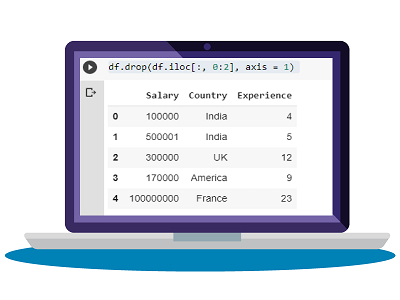# How to Drop One or More Columns in Python Pandas

Python Pandas allows you to easily work with data using different data types. Sometimes you may need to drop one or more columns in Python Pandas. In this article, we will look at the different ways to do this using Python pandas.

## How to Drop One or More Columns in Python Pandas

Let us say you have a simple dataframe that is a dictionary of lists with columns A, B, C, D, E. Here is a simple code to create this dataframe. We will first define a data dictionary and then use Python Pandas to convert it into dataframe.

```# Import pandas package
import pandas as pd

# create a dictionary with five fields each
data = {
'A':['A1', 'A2', 'A3', 'A4', 'A5'],
'B':['B1', 'B2', 'B3', 'B4', 'B5'],
'C':['C1', 'C2', 'C3', 'C4', 'C5'],
'D':['D1', 'D2', 'D3', 'D4', 'D5'],
'E':['E1', 'E2', 'E3', 'E4', 'E5'] }

# Convert the dictionary into DataFrame
df = pd.DataFrame(data)

print(df)```

Now we will look at different ways to drop columns in Python Pandas.

## 1. Using Drop() function

Once you have the dataframe, you can easily use drop() function to remove one or more columns from it as shown below. Here is the command to delete column A. We use axis=1 to delete columns. If you set it to 0 then it will delete rows.

```# Remove column name 'A'
df.drop(['A'], axis = 1)```

Here is the command to delete multiple columns B, C.

```# Remove two columns name is 'C' and 'D'
df.drop(['C', 'D'], axis = 1)```

## 2. Remove Columns based on Column Index

In the above commands, we have deleted columns using their column names. But sometimes your data may not have column names and you may need to delete columns based on their index. In such cases, you can use columns() function to specify the column index, instead of using column names. Please note, the column indexes start from 0, with the leftmost column having column index 0. Here is the command to delete columns with index 2, 4 in our data.

```# Remove two columns as index base
df.drop(df.columns[[2,4]], axis = 1, inplace = True)```

The above command will remove columns B & D.

Sometimes you may have many columns in your data and you may need to delete many columns from it. In such cases, it can be tedious to manually specify each column name or index to be deleted. If you want to delete a continuous range of columns, you can use iloc function. Here is an example to delete all columns between column index 1 and 3.

```# Remove all columns between column index 1 to 3
df.drop(df.iloc[:, 1:3], inplace = True, axis = 1)```

Sometimes it may be difficult to keep track of column index if you have too many columns in your data. In such cases, if you want to delete continuous columns between two columns, you can use ix() function. Here is the command to delete columns between columns B & D.

```# Remove all columns between column name 'B' to 'D'
df.drop(df.ix[:, 'B':'D'].columns, axis = 1)```

Similarly, you can also use locate() function to locate the appropriate columns, and drop() function to drop them. Here is the command to delete columns between columns B & D

```# Remove all columns between column name 'B' to 'D'
df.drop(df.loc[:, 'B':'D'].columns, axis = 1)```

## 3. Drop Columns Iteratively

Sometimes you may want to drop certain columns that satisfy or do not satisfy specific conditions. In such cases, you will need to iteratively delete columns, depending on whether they meet the criteria for deletion. Here is a simple way to loop through the columns of your dataframe and delete column A.

```for col in df.columns:
if 'A' in col:
del df[col]
```

In this article, we have learnt several ways to delete columns in Python dataframe and also learnt the specific use cases under which to use each method. You can modify the above code as per your requirement.